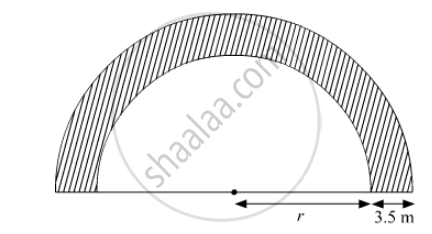Advertisement Remove all ads

# A Path of Width 3.5 M Runs Around a Semi-circular Grassy Plot Whose Perimeter is 72 M . Find the Area of the Path . ( Use π = 22 7 ) - Mathematics

Sum

A path of width 3.5 m  runs around a semi-circular grassy plot whose perimeter is 72 m . Find the area of the path .

("Use"  pi= 22/7)

Advertisement Remove all ads

#### SolutionLet the radius of the semicircular plot be r
Perimeter of the semi-circular grassy plot = pi r+2r=72

⇒ r=14 cm

Given that the width of the plot = 3.5 m
Thus, the outer radius = 3.5 + 14 = 17.5 m

Area of the path = (pi R^2)/2-(pi r^2)/2

= pi/2 (R^2-r^2)

=pi/2 ((17.5)^2-(14)^2)

= 173.25 m^2

Is there an error in this question or solution?
Advertisement Remove all ads

#### APPEARS IN

RD Sharma Class 10 Maths
Chapter 13 Areas Related to Circles
Exercise 13.1 | Q 27 | Page 13
Advertisement Remove all ads

#### Video TutorialsVIEW ALL 

Advertisement Remove all ads
Share
Notifications

View all notifications

Forgot password?
Course